# Top 20 Geometry Questions For CMAT

0
237

Geometry is an important topic that is included in the CMAT Exam, a national-level entrance exam for MBA aspirants in India. Geometry questions are designed to test the candidates’ understanding of shapes, figures, and spatial relationships. To help students prepare for the exam, we have compiled a list of the top 20 geometry questions for CMAT. These questions cover a range of concepts, from basic principles of geometry to more complex problem-solving techniques. By practicing these questions, students can improve their problem-solving skills and increase their chances of scoring well on the geometry section of the CMAT. Click on the link given below to download the top 20 Geometry questions for CMAT with detailed solutions PDF.

Question 1: The length of a rectangle is quadrupled and the breadth is made 1/5th of the original breadth. What is the percentage change in area of the rectangle ?

a) 50% decrease

b) 50% increase

c) 20% increase

d) 20% decrease

Solution:

Let the length of the rectangle be l and the breadth of the rectangle be b.
Original area = lb.
Area of the rectangle after making changes = 4l x $\frac{1}{5}b = 4/5 lb.$
Thus, decrease in area = lb – 4/5 lb = 1/5 lb
∴ percentage decrease in area = $\frac{\frac{1}{5} lb}{lb} \times100$% = 20%

Question 2: A room is 12m long and 8m broad. The area of the 4 walls of the room is 48m$^2$ more than the sum of areas of the floor and the ceiling. Find the volume of the room ?

a) 600m$^2$

b) 476m$^2$

c) 450m$^2$

d) 576m$^2$

Solution:

area of 4 walls = 2(lh) + 2(bh)
area of floor + ceiling = 2lb = 2x12x8 = 192m
Given, 2lh + 2bh = 192 + 48
12h + 8h = 120
thus, h = 6m
∴: the volume of the room = lxbxh = 12x8x6 = 576m$^2$

Question 3: Sunil Shetty cycles at 12 metres per second. How long will he take to cycle around a circular field of radius 45 metres?

a) 13.57 s

b) 23.57 s

c) 33.57 s

d) 43.57 s

Solution:

The perimeter of the circle is 2 x $\pi$ x 45 = 2 x 3.14 x 45 = 282.86 m
Time = distance/ speed = 282.86 /12 = 23.57 seconds

Question 4: In the figure below, AC||DE. Find AD.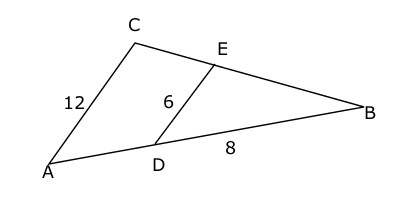a) 12 cm

b) 9 cm

c) 8 cm

d) 7 cm

Solution:

Since AC||DE, $\angle D = \angle A, \angle E = \angle C$
Therefore ABC ~ DBE.

Question 5: In the diagram below, PR || TQ, and TP = 18 cm, PS = 6 cm, QR = 8cm and TQ = 10 cm. Find perimeter of triangle PRS.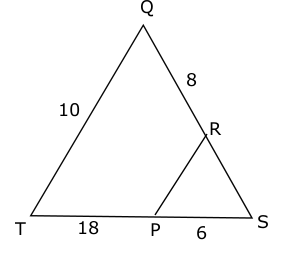a) 16.14 cm

b) 11.16 cm

c) 8.3 cm

d) 14.66 cm

Solution:

Since TQ||RP, $\angle R = \angle Q, \angle P = \angle T$
==> Triangle PRS ~ TQS.
==>SP/ST = PR/TQ ==> 1/4 = PR/10 ==> PR = 2.5 cm.
Similarly, RS = 8/3.
Therefore perimeter of triangle PRS = 6+2.5+2.66=11.16cm.

Question 6: In the diagram below, DC || FB, FE||BC, AB = 12cm and FE =BC/2. DC=?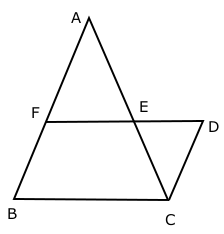a) $4.5$ cm

b) $6$ cm

c) $8$ cm

d) $4\sqrt3$ cm

Solution:

Given FE||BC ==> triangles AFE, ABC are similar ==> FE/BC = AF/AB ==> AF=AB/2=FB

Also given FB||DC ==> FDCB is parallelogram ==> DC =FB = 6 cm.
So the correct option to choose is B – 6cm.

Question 7: In the given figure AB is parallel to CD and XY is a transversal as shown. If Angle XEA = 100 degrees, find the value of angle XFD?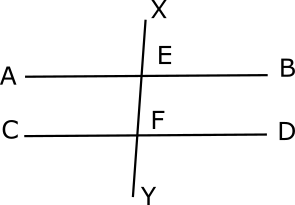a) 60 degrees

b) 70 degrees

c) 80 degrees

d) 90 degrees

Solution:

Since Angle XEA and XFC are corresponding angles,
Angle XEA = Angle XFC = 100 degrees
Angle XFC + Angle XFD = 180 degrees
Angle XFD = 180-100 = 80 degrees

Question 8: In the given figure AB is parallel to CD and XY is a transversal as shown. If Angle XEB = 110 degrees, find the value of Angle DFY?a) 70 degrees

b) 80 degrees

c) 90 degrees

d) 100 degrees

Solution:

Since the sum of exterior angles on the same side of the transversal is equal to 180 degrees,

Angle XEB + Angle DFY = 180 degrees

110 + Angle DFY = 180

Angle DFY = 180-110 = 70 degrees

Question 9: The volume of a cylinder is $13122 \pi\text{ }cm^{3}$. The ratio between the slant height of cone to the height of cylinder is 5:3 respectively. If the radius of cylinder is 50% more than the height of cylinder and the total surface area of cone is $1296 \pi\text{ }cm^{2}$, then find out the height of cone.

a) 27 cm

b) 24 cm

c) 18 cm

d) 30 cm

e) None of the above

Solution:

The volume of a cylinder is $13122 \pi\text{ }cm^{3}$.
$\pi\times (radius_{cylinder})^{2}\times (height_{cylinder}) = 13122 \pi$
$(radius_{cylinder})^{2}\times (height_{cylinder}) = 13122$
If the radius of cylinder is 50% more than the height of cylinder.
Let’s assume the height of cylinder is 2y
radius of cylinder = 150% of 10y = 3y
$(3y)^{2}\times (2y) = 13122$
$9y^{2}\times 2y = 13122$
$18y^{3} = 13122$
$y^{3} = 729$
$y^{3} = 9^{3}$
y = 9
height of cylinder = 2y
= $2\times9$
= 18 cm
= $3\times9$
= 27 cm
The ratio between the slant height of cone to the height of cylinder is 5:3 respectively.
slant height of cone = $\frac{18}{3}\times5$
= 30 cm
Total surface area of cone is $1296 \pi\text{ }cm^{2}$.
$\pi\times radius\times(radius+\text{slant height of cone}) = 1296 \pi$
$radius \times(radius+30) = 1296$
$(radius)^{2} + 30radius = 1296$
$(radius)^{2} + 30radius – 1296 = 0$
$(radius)^{2} + (54-24)radius – 1296 = 0$
$(radius)^{2} + 54radius – 24radius – 1296 = 0$
height of cone = $\sqrt{(30)^{2}-(24)^{2}}$
= $\sqrt{900-576}$
= $\sqrt{324}$
= 18 cm
Hence, option c is the correct answer.

Question 10: The area of circle P is 1694 $cm^{2}$ more than the area of circle Q. The perimeter of a rectangle is 154 cm whose length and breadth are equal to the radius of circle P and Q respectively. Find out the area of rectangle.

a) 1290 $cm^{2}$

b) 1640 $cm^{2}$

c) 1580 $cm^{2}$

d) 1360 $cm^{2}$

e) None of the above

Solution:

Let’s assume the radius of circle P and Q are $R_{p}$ and $R_{q}$ respectively.
The area of circle P is 1694 $cm^{2}$ more than the area of circle Q.
$\pi \times (R_{p})^{2} = \pi \times (R_{q})^{2}+1694$
$\frac{22}{7}\times (R_{p})^{2} = \frac{22}{7}\times (R_{q})^{2}+1694$
$\frac{1}{7}\times (R_{p})^{2} = \frac{1}{7}\times (R_{q})^{2}+77$
$(R_{p})^{2}-(R_{q})^{2} = 539$
$(R_{p}-R_{q}) (R_{p}+R_{q}) = 539$ Eq.(i)
The perimeter of a rectangle is 154 cm whose length and breadth are equal to the radius of circle P and Q respectively.
$2(R_{p}+R_{q}) = 154$
$R_{p}+R_{q} = 77$ Eq.(ii)
Put Eq.(ii) in Eq.(i).
$77(R_{p}-R_{q}) = 539$
$R_{p}-R_{q} = 7$ Eq.(iii)
$2R_{p} = 77+7$
$2R_{p} = 84$
$R_{p} = 42$ Eq.(iv)
Put Eq.(iv) in Eq.(iii).
$42-R_{q} = 7$
$R_{q} = 42-7 = 35$
area of rectangle = $R_{p} \times R_{q}$
= $42\times35$
= 1470 $cm^{2}$
Hence, option e is the correct answer.

Question 11: In the given figure, the area of the unshaded region is 35% of the area of the shaded region. What is the value of x?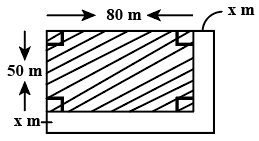a) 20

b) 15

c) 10

d) 5

Question 12: The area of a trapezium, whose parallel sides are 25 cm and 19 cm long, is 330 $cm^{2}$. The distance between the sides (in cm) is:

a) 10

b) 12

c) 30

d) 15

Question 13: The perimeter and area of a rectangular sheet are 94 m and 420 $m^{2}$ respectively. The length of the diagonal will be:

a) 32 m

b) 36 m

c) 35 m

d) 37 m

Question 14: The sum of the radius of the base and slant height of a right circular cone is 49 cm. If the total surface area is 1848 $cm^{2}$, then its height is:
(Take $\pi=\frac{22}{7}$)

a) 36 cm

b) 30 cm

c) 32 cm

d) 35 cm

Question 15: The radius of a cylinder is increased by 20% and its height is decreased by 45%. What is the percentage increase/decrease in the volume of the cylinder?

a) Increase, 25%

b) Decrease 20.8%

c) Decrease, 25%

d) Increase, 20.8%

Question 16: The length and breadth of a rectangle are increased by 25% and 32%, respectively. The percentage increase in the area of the resulting rectangle will be:

a) 65%

b) 60%

c) 62%

d) 64%

Question 17: The area of a triangular park with sides 78 m, 160 m and 178 m is equal to the area of a rectangular garden whose sides are in the ratio of 13 : 12. The smaller side (in m) of the garden is:

a) $26\sqrt{5}$

b) $24\sqrt{10}$

c) $12\sqrt{10}$

d) $13\sqrt{5}$

Question 18: If the radius of a sphere is increased by $16\frac{2}{3}\%$, then by what per cent would its surface area increase (correct to one decimal place)

a) 34.8%

b) 36.1%

c) 33.9%

d) 35.6%

Question 19: A solid metallic toy has a hemispherical base with radius 3.5 cm surmounted by a cone. If the height of the cone is the same as the radius of its base, then the volume of metal used in $cm^{3}$ is: (Take $\pi=\frac{22}{7}$)

a) $89\frac{2}{3}$ $cm^{3}$

b) $144\frac{3}{4}$ $cm^{3}$

c) $134\frac{3}{4}$ $cm^{3}$

d) $179\frac{2}{3}$ $cm^{3}$